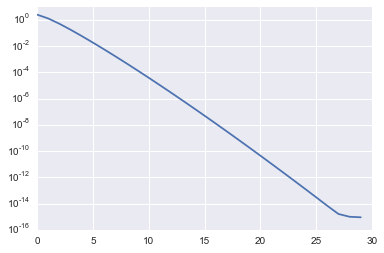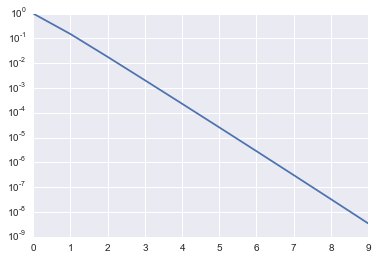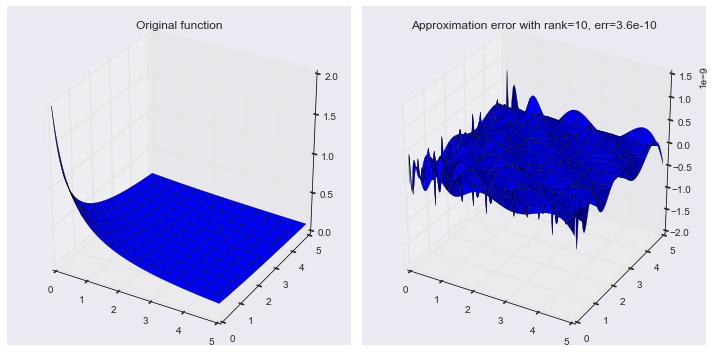# Lecture 6: Matrix rank, singular value decomposition¶

## Syllabus¶

Week 1: Matrices, vectors, matrix/vector norms, scalar products & unitary matrices
Week 2: TAs-week (Strassen, FFT, a bit of SVD)
Week 3: Matrix ranks, singular value decomposition, linear systems

## Recap of the previous lecture (a week ago)¶

• Concept of block matrices (Lecture 3.1)
• Matrix norms, scalar products, unitary matrices (Lecture 3.2)

## Today lecture¶

• Rank of the matrix, idea of linear dependence
• Singular value decomposition (SVD) and low-rank approximation

## Matrix and linear spaces¶

A matrix can be considered as a sequence of vectors, its columns: $$A = [a_1, \ldots, a_m],$$ where $a_m \in \mathbb{C}^n$.
A matrix-by-vector product is equivalent to taking a linear combination of those columns
$$y = Ax, \quad \Longleftrightarrow y = a_1 x_1 + a_2 x_2 + \ldots +a_m x_m.$$

This is a special case of block matrix notation that we have already seen.

## Linear dependence¶

The vectors are called linearly dependent, if there exist non-zero coefficients $x_i$ such that
$$\sum_i a_i x_i = 0,$$ or in the matrix form, $$Ax = 0, \quad \Vert x \Vert \ne 0.$$ In this case, we say that the matrix $A$ has a non-trivial nullspace.

## Dimension of a linear space¶

A linear space is defined as all possible vectors of the form $$\sum_{i=1}^m a_i x_i,$$ where $x_i$ are some coefficients and $a_i, i = 1, \ldots, m$ are given vectors. In the matrix form, the linear space is a collection of the vectors of the form
$$A x.$$ This set is also called the range of the matrix.

## Matrix rank¶

What is a matrix rank?

A matrix rank is defined as the maximal number of linearly independent columns in a matrix, or the dimension of its column space.

You can also use linear combination of rows to define the rank.

Theorem
The dimension of the column space of the matrix is equal to the dimension of the row space of the matrix.

## Are there any "small-dimensional subspaces"?¶

• Of course, not! (I.e. a random matrix is a full-rank matrices).

Why do we care?

## In practice, everything is "small-dimensional"¶

Johnson–Lindenstrauss lemma

Given $0 < \epsilon < 1$, a set of points in $\mathbb{R}^N$ and $n > \frac{8 \log m}{\epsilon^2}$

and linear map $f$ from $\mathbb{R}^N \rightarrow \mathbb{R}^M$

$$(1 - \epsilon) \Vert u - v \Vert^2 \leq \Vert f(u) - f(v) \Vert^2 \leq (1 + \epsilon) \Vert u - v \Vert^2.$$

## Skeleton decomposition¶

A very useful representation for computation of the matrix rank is the skeleton decomposition, which can be graphically represented as follows:or in the matrix form $$A = U \widehat{A}^{-1} V^{\top},$$ where $U$ are some $r$ columns of $A$, $V^{\top}$ are some rows of $A$, $\widehat{A}$ is the submatrix on the intersection, which should be nonsingular.

Remark.
An inverse of the matrix $P$ the matrix $Q = P^{-1}$ such that
$P Q = QP = I$.
If the matrix is square and has full rank (i.e. equal to $n$) then the inverse exists.

## Proof for the skeleton decomposition¶

Let $U$ be the $r$ columns based on the submatrix $\widehat{A}$. Then they are linearly independent. Take any other column $u$ of $A$. Then it is a linear combination of the columns of $U$, i.e.
$u = U x$.
These are $n$ equations. We take $r$ of those corresponding to the rows that contain $\widehat{A}$ and get the equation
$$\widehat{u} = \widehat{A} x,$$ therefore
$$x = \widehat{A}^{-1} \widehat{u},$$ and that gives us the result.

## A closer look¶

Any rank-$r$ matrix can be written in the form $$A = U \widehat{A}^{-1} V^{\top},$$ where $U$ is $n \times r$, $V$ is $m \times r$ and $\widehat{A}$ is $r \times r$, or $$A = U' V'^{\top}.$$ So, every rank-$r$ matrix can be written as a product of a "tall" matrix $U'$ by a long matrix $V'$.

In the index form, it is
$$a_{ij} = \sum_{\alpha=1}^r u_{i \alpha} v_{j \alpha}.$$ For rank 1 we have $$a_{ij} = u_i v_j,$$ i.e. it is a separation of indices and rank-$r$ is a sum of rank-$1$ matrices!

### Storage¶

It is interesting to note, that for the rank-$r$ matrix $$A = U V^{\top}$$ only $U$ and $V$ can be stored, which gives us $(n+m) r$ parameters, so it can be used for compression. We can also compute matrix-by-vector product much faster.

In :
import numpy as np
n = 1000
r = 10
u = np.random.randn(n, r)
v = np.random.randn(n, r)
a = u.dot(v.T)
x = np.random.randn(n)
%timeit a.dot(x)
%timeit u.dot(v.T.dot(x))

The slowest run took 6.03 times longer than the fastest. This could mean that an intermediate result is being cached
1000 loops, best of 3: 562 µs per loop
The slowest run took 6.92 times longer than the fastest. This could mean that an intermediate result is being cached
100000 loops, best of 3: 12.8 µs per loop


We can also try to compute the matrix rank using the built-in np.linalg.matrix_rank function

In :
#Computing matrix rank
import numpy as np
n = 50
a = np.ones((n, n))
print 'Rank of the matrix:', np.linalg.matrix_rank(a)
b = a + 1e-6 * np.random.randn(n, n)
print 'Rank of the matrix:', np.linalg.matrix_rank(b, tol=1e-8)

Rank of the matrix: 1
Rank of the matrix: 50


### Instability of the matrix rank¶

For any rank-$r$ matrix $A$ with $r < \min(m, n)$ there is a matrix $B$ such that its rank rank is equal to $\min(m, n)$ and $$\Vert A - B \Vert = \varepsilon.$$ So, does this mean that numerically matrix rank has no meaning? (I.e., small perturbations lead to full rank)!
The solution is to compute the best rank-r approximation to a matrix.

## Singular value decomposition¶

To compute low-rank approximation, we need to compute singular value decomposition.
Any matrix $A$ can be written as a product of three matrices:
$$A = U \Sigma V^*,$$ where $U$ is an $n \times K$ unitary matrix, $V$ is an $m \times K$ unitary matrix, $K = \min(m, n)$ $\Sigma$ is a diagonal matrix with non-negative elements $\sigma_1 \geq \ldots, \geq \sigma_K$ on the diagonal.

Computation of the best rank-$r$ approximation is equivalent to setting $\sigma_{r+1}= 0, \ldots, \sigma_K = 0$. The error is then determined by the omitted singular value!
$$\min_{A_r} \Vert A - A_r \Vert = \sigma_{r+1},$$ that is why it is important to look at the decay of the singular vectors.

## Low-rank approximation via SVD¶

$\Vert A - A_r \Vert = \Vert U \Sigma V^* - A_r \Vert = \Vert \Sigma - U^* A_r V \Vert$,
where we used that for spectral norm (and for the Frobenius as well) we have

$$\Vert X Q \Vert = \Vert X \Vert$$

for any unitary $Q$ and any matrix $X$. This is called unitary equivalence of the spectral norm (and Frobenius as well).
What is left is to note that the best rank-$r$ approximation of the diagonal matrix is its subdiagonal.

Computing the SVD is tricky; we will talk about the algorithms hidden in this computation later. But we already can do this in Python!

In :
#Computing matrix rank
import numpy as np
n = 50
a = np.ones((n, n))
print 'Rank of the matrix:', np.linalg.matrix_rank(a)
b = a + 1e-5 * np.random.randn(n, n)
print 'Rank of the matrix:', np.linalg.matrix_rank(b)

Rank of the matrix: 1
Rank of the matrix: 50

In :
u, s, v = np.linalg.svd(b)
print s/s
r = 1
u1 = u[:, :r]
s1 = s[:r]
v1 = v[:r, :]
a1 = u1.dot(np.diag(s1).dot(v1))
print np.linalg.norm(b - a1, 2)/s

2.62173054286e-06
2.62173054287e-06


We can use SVD to compute approximations of function-related matrices, i.e. the matrices of the form $$a_{ij} = f(x_i, y_j),$$ where $f$ is a certain function, and $x_i, \quad i = 1, \ldots, n$ and $y_j, \quad j = 1, \ldots, m$ are some one-dimensional grids.

In :
%matplotlib inline
import numpy as np
import matplotlib.pyplot as plt
import seaborn as sns
n = 1000
a = [[1.0/(i+j+1) for i in xrange(n)] for j in xrange(n)] #Hilbert matrix
a = np.array(a)
u, s, v = np.linalg.svd(a)
fig, ax = plt.subplots(1, 1)
ax.semilogy(s[:30])
#We have very good low-rank approximation of it!

Out:
[<matplotlib.lines.Line2D at 0x11ec0f150>]Now we can do something more interesting, like function approximation

In :
import numpy as np
n = 128
t = np.linspace(0, 5, n)
x, y = np.meshgrid(t, t)
f = 1.0 / (x + y + 0.5)
u, s, v = np.linalg.svd(f, full_matrices=False)
r = 10
u = u[:, :r]
s = s[:r]
v = v[:r, :] #Mind the transpose here!
fappr = u.dot(np.diag(s).dot(v))
er = np.linalg.norm(fappr - f, 'fro') / np.linalg.norm(f, 'fro')
print er
plt.semilogy(s/s)

3.61306034376e-10

Out:
[<matplotlib.lines.Line2D at 0x11f5dcf90>]And do some 3D plotting

In :
%matplotlib inline
import matplotlib.pyplot as plt
from mpl_toolkits.mplot3d import Axes3D
fig = plt.figure(figsize=(10, 5))
ax.plot_surface(x, y, f)
ax.set_title('Original function')
ax.plot_surface(x, y, fappr - f)
ax.set_title('Approximation error with rank=%d, err=%3.1e' % (r, er))
fig.tight_layout()## Linear factor analysis & low-rank¶

Consider a linear factor model,

$$y = Ax,$$

where $y$ is a vector of length $n$, and $x$ is a vector of length $r$.
The data is organizes as samples: we observe vectors
$$y_1, \ldots, y_T,$$ then the factor model can be written as
$$Y = AX,$$ where $Y$ is $n \times T$, $A$ is $n \times r$ and $X$ is $r \times T$. This is exactly a rank-$r$ model: it tells us that the vector lie in a small subspace!
We also can use SVD to recover this subspace (but not the independent components). Principal component analysis can be done by SVD!

## Take home message¶

• Matrix rank definition
• Skeleton approximation and dyadic representation of a rank-$r$ matrix
• Singular value decomposition

## Next lecture¶

• Linear systems
• Inverse matrix
• Condition number
• Linear least squares
• Pseudoinverse
##### Questions?¶
In :
from IPython.core.display import HTML
def css_styling():